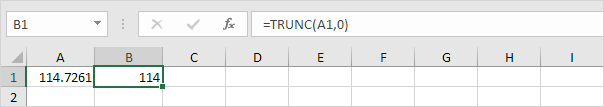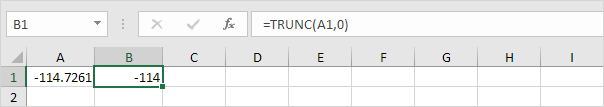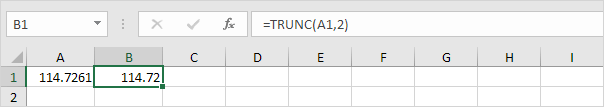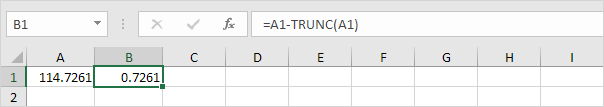# Chop off Decimals

This example illustrates two functions to chop off decimals in Excel. INT and TRUNC.

### Int

The INT (Integer) function rounds a number down to the nearest integer (negative numbers become more negative).Conclusion: the INT function only chops off decimals from positive numbers.

### Trunc

The TRUNC (Truncate) function always chops off decimals.The TRUNC function can keep any number of decimals. Simply use the second argument to specify how many decimals you want to keep.### Decimal Part

To return just the decimal part of a number, use the following formula.Note: if the second argument of the TRUNC function is omitted, a value of 0 is assumed.

Go to Next Chapter: Formula Errors